# 6. Discretized Non-negative Tensor Factorization (dNTF)

Laboratory for Bioinformatics Research, RIKEN Center for Biosystems Dynamics Research

# Introduction

In this vignette, we consider approximating a non-negative tensor as a product of binary or non-negative low-rank matrices (a.k.a., factor matrices).

Test data is available from toyModel.

library("dcTensor")
X <- dcTensor::toyModel("dNTF")

You will see that there are four blocks in the data tensor as follows.

suppressMessages(library("nnTensor"))
plotTensor3D(X)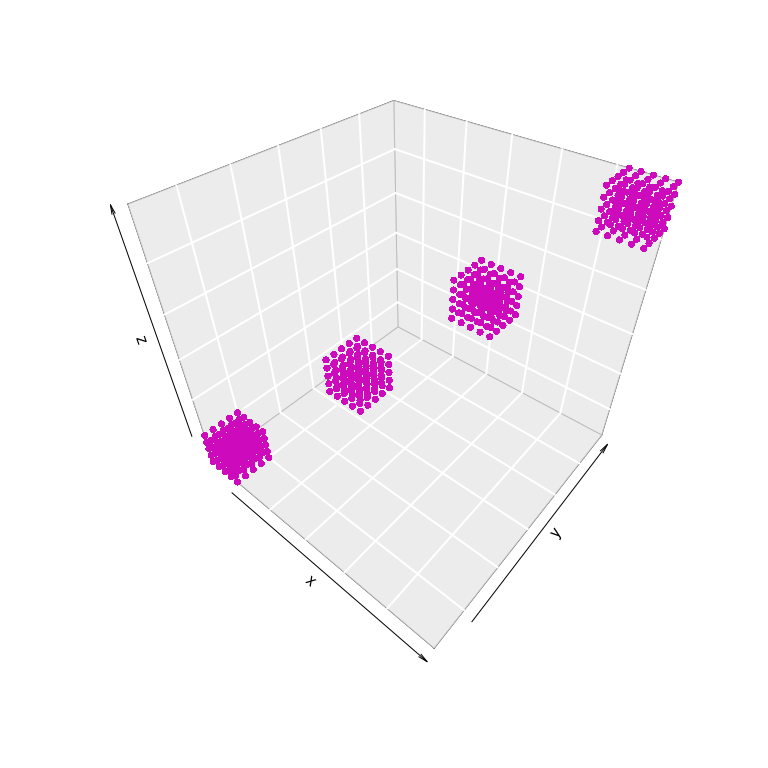# Binary Tensor Factorization (BTF)

To decompose a binary tensor ($$\mathcal{X}$$), non-negative CP decomposition (a.k.a. non-negative tensor factorization; NTF (Cichocki 2007; CICHOCK 2009)) can be applied. NTF appoximates $$\mathcal{X}$$ ($$N \times M \times L$$) as the mode-product of a core tensor $$S$$ ($$J \times J \times J$$) and factor matrices $$A_1$$ ($$J \times N$$), $$A_2$$ ($$J \times M$$), and $$A_3$$ ($$J \times L$$).

$\mathcal{X} \approx \mathcal{S} \times_{1} A_1 \times_{2} A_2 \times_{3} A_3\ \mathrm{s.t.}\ \mathcal{S} \geq 0, A_{k} \geq 0\ (k=1 \ldots 3)$

Note that _{k} is the mode-$$k$$ product (CICHOCK 2009) and the core tensor $$S$$ has non-negative values only in the diagonal element. For the details, see NTF function of nnTensor package.

## Basic Usage

In BTF, a rank parameter $$J$$ ($$\leq \min(N, M)$$) is needed to be set in advance. Other settings such as the number of iterations (num.iter) or factorization algorithm (algorithm) are also available. For the details of arguments of dNTF, see ?dNTF. After the calculation, various objects are returned by dNTF. BTF is achieved by specifying the binary regularization parameter as a large value like the below:

set.seed(123456)
out_dNTF <- dNTF(X, Bin_A=c(1e+2, 1e+2, 1e+2), algorithm="KL", rank=4)
str(out_dNTF, 2)
## List of 6
##  $S : num [1:4] 2.24 2.23 2.24 2.24 ##$ A            :List of 3
##   ..$: num [1:4, 1:30] 9.99e-01 2.22e-16 2.22e-16 1.00 9.99e-01 ... ## ..$ : num [1:4, 1:30] 1.00 2.22e-16 2.22e-16 2.22e-16 1.00 ...
##   ..$: num [1:4, 1:30] 4.47e-01 9.94e-17 9.93e-17 9.93e-17 4.47e-01 ... ##$ RecError     : Named num [1:28] 1.00e-09 2.67e+01 2.45e+01 2.36e+01 2.27e+01 ...
##   ..- attr(*, "names")= chr [1:28] "offset" "1" "2" "3" ...
##  $TrainRecError: Named num [1:28] 1.00e-09 2.67e+01 2.45e+01 2.36e+01 2.27e+01 ... ## ..- attr(*, "names")= chr [1:28] "offset" "1" "2" "3" ... ##$ TestRecError : Named num [1:28] 1e-09 0e+00 0e+00 0e+00 0e+00 0e+00 0e+00 0e+00 0e+00 0e+00 ...
##   ..- attr(*, "names")= chr [1:28] "offset" "1" "2" "3" ...
##  $RelChange : Named num [1:28] 1.00e-09 2.56e-02 8.80e-02 4.16e-02 3.89e-02 ... ## ..- attr(*, "names")= chr [1:28] "offset" "1" "2" "3" ... The reconstruction error (RecError) and relative error (RelChange, the amount of change from the reconstruction error in the previous step) can be used to diagnose whether the calculation is converged or not. layout(t(1:2)) plot(log10(out_dNTF$RecError[-1]), type="b", main="Reconstruction Error")
plot(log10(out_dNTF$RelChange[-1]), type="b", main="Relative Change")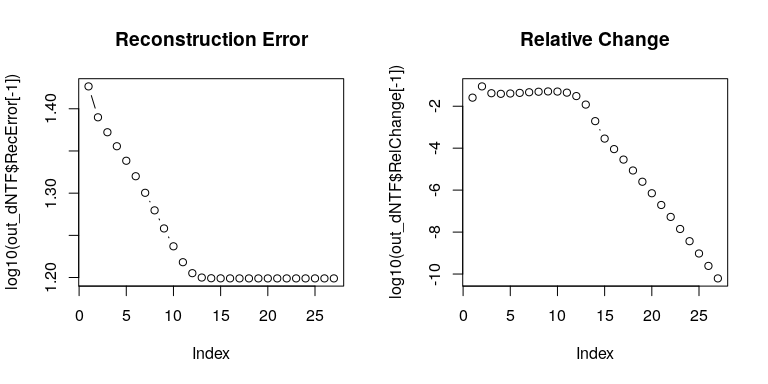The product of core tensor $$S$$ and factor matrices $$A_{k}$$ shows whether the original data is well-recovered by dNTF. recX <- recTensor(out_dNTF$S, out_dNTF$A) layout(t(1:2)) plotTensor3D(X) plotTensor3D(recX, thr=0)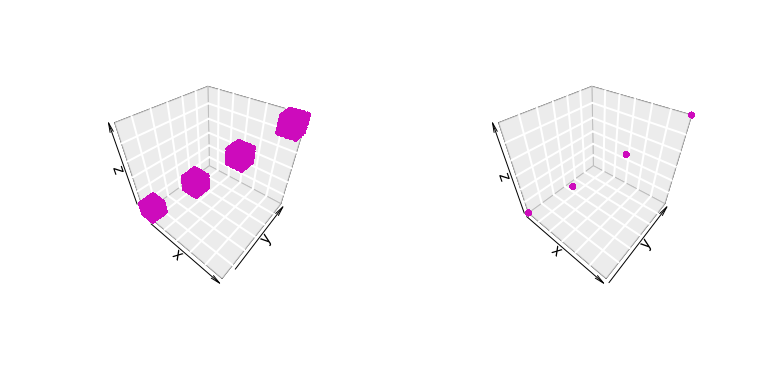The histograms of $$A_{k}$$s show that all the factor matrices $$A_{k}$$ looks binary. layout(t(1:3)) hist(out_dNTF$A[], main="A1", breaks=100)
hist(out_dNTF$A[], main="A2", breaks=100) hist(out_dNTF$A[], main="A3", breaks=100)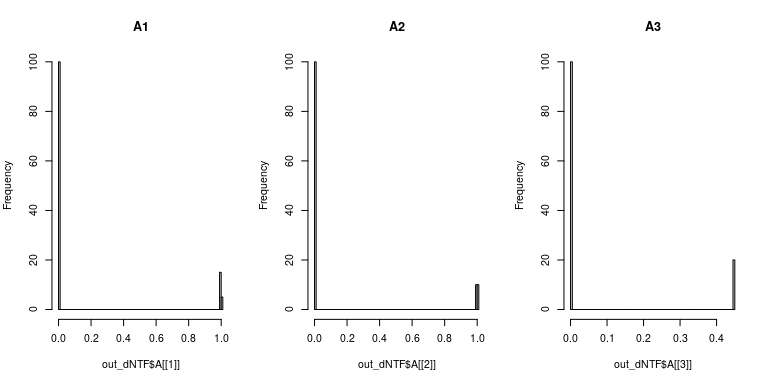# Semi-Binary Tensor Factorization (SBTF)

Here, we define this formalization as semi-binary tensor factorization (SBTF). SBTF can capture discrete patterns from non-negative matrices.

To demonstrate SBMF, next we use a non-negative tensor from the nnTensor package. You will see that there are four blocks in the data tensor as follows.

X2 <- nnTensor::toyModel("CP")
plotTensor3D(X2)## Basic Usage

In SBTF, a rank parameter $$J$$ ($$\leq \min(N, M)$$) is needed to be set in advance. Other settings such as the number of iterations (num.iter) or factorization algorithm (algorithm) are also available. For the details of arguments of dNTF, see ?dNTF. After the calculation, various objects are returned by dNTF. SBTF is achieved by specifying the binary regularization parameter as a large value like the below:

set.seed(123456)
out_dNTF2 <- dNTF(X2, Bin_A=c(1e+5, 1e+5, 1e-10), algorithm="KL", rank=4)
str(out_dNTF2, 2)
## List of 6
##  $S : num [1:4] 13.1 31.7 112.1 1474.1 ##$ A            :List of 3
##   ..$: num [1:4, 1:30] 0.00704 0.00175 0.47548 0.00303 0.00653 ... ## ..$ : num [1:4, 1:30] 0.00905 0.00602 0.10119 0.00226 0.0092 ...
##   ..$: num [1:4, 1:30] 0.1385 0.2206 0.0447 0.0048 0.1523 ... ##$ RecError     : Named num [1:101] 1.00e-09 2.46e+04 3.90e+03 2.96e+03 6.38e+03 ...
##   ..- attr(*, "names")= chr [1:101] "offset" "1" "2" "3" ...
##  $TrainRecError: Named num [1:101] 1.00e-09 2.46e+04 3.90e+03 2.96e+03 6.38e+03 ... ## ..- attr(*, "names")= chr [1:101] "offset" "1" "2" "3" ... ##$ TestRecError : Named num [1:101] 1e-09 0e+00 0e+00 0e+00 0e+00 0e+00 0e+00 0e+00 0e+00 0e+00 ...
##   ..- attr(*, "names")= chr [1:101] "offset" "1" "2" "3" ...
##  $RelChange : Named num [1:101] 1.00e-09 8.23e-01 5.30 3.19e-01 5.36e-01 ... ## ..- attr(*, "names")= chr [1:101] "offset" "1" "2" "3" ... RecError and RelChange can be used to diagnose whether the calculation is converged or not. layout(t(1:2)) plot(log10(out_dNTF2$RecError[-1]), type="b", main="Reconstruction Error")
plot(log10(out_dNTF2$RelChange[-1]), type="b", main="Relative Change")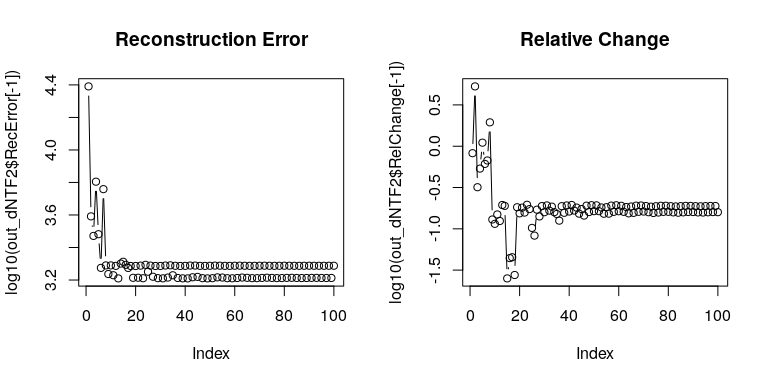The product of core tensor $$S$$ and factor matrices $$A_{k}$$ shows whether the original data is well-recovered by dNTF. recX <- recTensor(out_dNTF2$S, out_dNTF2$A) layout(t(1:2)) plotTensor3D(X2) plotTensor3D(recX, thr=0)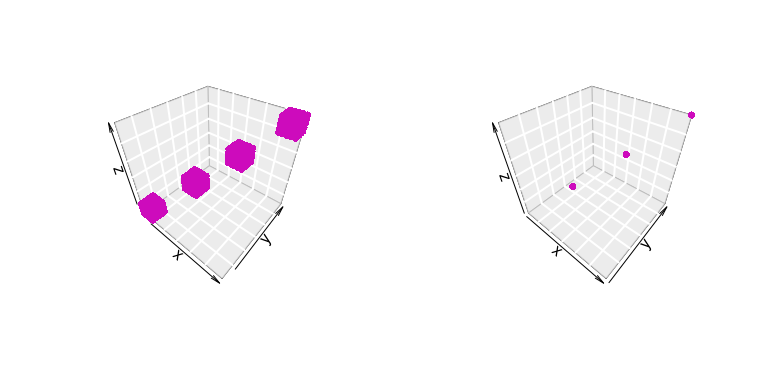The histograms of $$A_{k}$$s show that $$A_{k}$$ looks binary. layout(t(1:3)) hist(out_dNTF2$A[], main="A1", breaks=100)
hist(out_dNTF2$A[], main="A2", breaks=100) hist(out_dNTF2$A[], main="A3", breaks=100)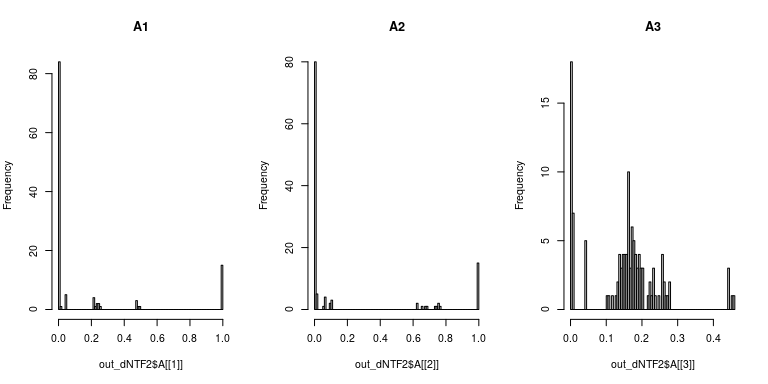# Session Information

## R version 4.3.0 (2023-04-21)
## Platform: x86_64-pc-linux-gnu (64-bit)
## Running under: Ubuntu 22.04.2 LTS
##
## Matrix products: default
## LAPACK: /usr/lib/x86_64-linux-gnu/openblas-pthread/libopenblasp-r0.3.20.so;  LAPACK version 3.10.0
##
## locale:
##   LC_CTYPE=en_US.UTF-8       LC_NUMERIC=C
##   LC_TIME=en_US.UTF-8        LC_COLLATE=C
##   LC_MONETARY=en_US.UTF-8    LC_MESSAGES=en_US.UTF-8
##   LC_PAPER=en_US.UTF-8       LC_NAME=C
##  LC_MEASUREMENT=en_US.UTF-8 LC_IDENTIFICATION=C
##
## time zone: Etc/UTC
## tzcode source: system (glibc)
##
## attached base packages:
##  stats     graphics  grDevices utils     datasets  methods   base
##
## other attached packages:
##  nnTensor_1.1.13   fields_14.1       viridis_0.6.3     viridisLite_0.4.2
##  spam_2.9-1        dcTensor_1.2.0
##
## loaded via a namespace (and not attached):
##   gtable_0.3.3       jsonlite_1.8.5     highr_0.10         dplyr_1.1.2
##   compiler_4.3.0     maps_3.4.1         Rcpp_1.0.10        tagcloud_0.6
##   plot3D_1.4         tidyselect_1.2.0   gridExtra_2.3      jquerylib_0.1.4
##  scales_1.2.1       yaml_2.3.7         fastmap_1.1.1      ggplot2_3.4.2
##  R6_2.5.1           tcltk_4.3.0        generics_0.1.3     knitr_1.43
##  MASS_7.3-60        misc3d_0.9-1       dotCall64_1.0-2    tibble_3.2.1
##  munsell_0.5.0      RColorBrewer_1.1-3 bslib_0.5.0        pillar_1.9.0
##  rlang_1.1.1        utf8_1.2.3         cachem_1.0.8       xfun_0.39
##  sass_0.4.6         cli_3.6.1          magrittr_2.0.3     digest_0.6.31
##  grid_4.3.0         rTensor_1.4.8      lifecycle_1.0.3    vctrs_0.6.2
##  evaluate_0.21      glue_1.6.2         fansi_1.0.4        colorspace_2.1-0
##  rmarkdown_2.22     tools_4.3.0        pkgconfig_2.0.3    htmltools_0.5.5